# Physical Chemistry : Boiling Point

## Example Questions

### Example Question #1 : Colligative Properties

What is the boiling point of a 100mL solution of water after the addition of 29g of sodium chloride?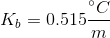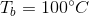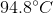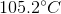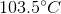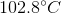Explanation:

We can use the boiling point elevation equation in order to find the new boiling point for the solution.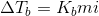The change in temperature will be equal to the boiling point elevation constant multiplied by the molality of the solution multiplied by the van't Hoff factor. The van't Hoff factor is a variable that incorporates how many ions the added solute will dissolve into when in solution. Sodium chloride will dissolve into two ions, so the van't Hoff factor is 2.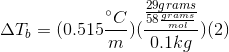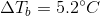This means that the boiling point of the water has been elevated by 5.2 degrees, meaning that the boiling point of the solution is 105.2 degrees celsius.

### All Physical Chemistry Resources